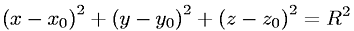Equations > Geometry > Curves and Shapes > Equation of a Sphere

### Equation of a SphereLatex Code:

MathML Code:

 ${\left(x-{x}_{0}\right)}^{2}+{\left(y-{y}_{0}\right)}^{2}+{\left(z-{z}_{0}\right)}^{2}={R}^{2}$

MathType 5.0: